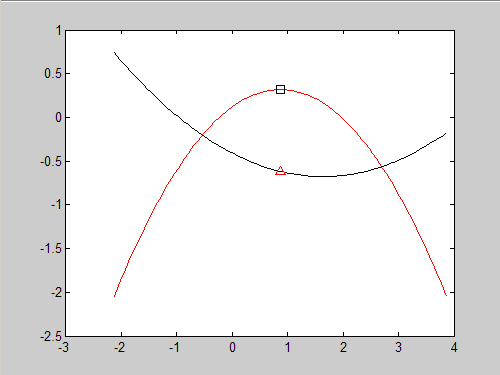# Puzzler: Working with polynomials

This puzzler is very straightforward, so I hope to hear from some of the newer MATLAB users. I think the solution is very linear, most people would come up with the same solution. As a hint, be sure to look at our help for POLYDER and ROOTS.

The challenge is this: Given the coefficients of two second order polynomials, find the local extrema of the first one. Knowing the X where this point happens, put a marker on both curves at that X value. Plot both curves for three units to the left and right of the inflection point of the first curve. [Edited Aug 27th in response to J. Paul R’s observation that I misused terminology, switching inflection point for local extrema. MATLAB t-shirt for the catch. Thank you!]Here is the code to create the coefficients:


coef1 = rand(1,3)-0.5;
coef2 = rand(1,3)-0.5;



<pre> <code>

all the code so someone can just copy and paste it from the comments.

</code> </pre>

|